Log inorRegisteror

### Kutools for Excel 22.00HOT

#### 300+ Powerful Features You Must Have in ExcelKutools for Excel is a powerful add-in that frees you from performing time-consuming operations in Excel, such as combine sheets quickly, merge cells without losing data, paste to only visible cells, count cells by color and so on. 300+ powerful features / functions for Excel 2019, 2016, 2013, 2010, 2007 or Office 365!

### Office Tab 14.00HOT

#### Adding Tabbed Interface for Office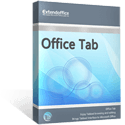It enables tabbed browsing, editing, and managing of Microsoft Office applications. You can open multiple documents / files in a single tabbed window, such as using the browser IE 8/9/10, Firefox, and Google Chrome. It's compatible with Office 2019, 2016, 2013, 2010, 2007, 2003 or Office 365. Demo

### Kutools for Outlook 13.00NEW

#### 100+ Powerful Features for OutlookKutools for Outlook is a powerful add-in that frees you from time-consuming operations which majority of Outlook users has to perform daily! It can save your time from using Microsoft Outlook 2019, 2016, 2013, 2010 or Office 365!

### Kutools for Word  9.00NEW

#### 100+ Powerful Features for WordKutools for Word is a powerful add-in that frees you from time-consuming operations which majority of Word users have to perform daily! It can save your time from using Microsoft Word / Office 2019, 2016, 2013, 2010, 2007, 2003 or Office 365!Restores the old look and menus of Office 2003 to Microsoft Office 2019, 2016, 2013, 2010, 2007 or Office 365. Don’t lose time in finding commands on the new Ribbon. Easy to deploy to all computers in enterprises and organizations.

## How to generate all prime numbers between two given numbers in Excel?

In Excel, have you ever tried to list all prime numbers between two given numbers? This article, I will talk about some interesting methods to deal with this task.

Generate all prime numbers between two given numbers with formulas

Generate all prime numbers between two given numbers with User Defined Function

####Generate all prime numbers between two given numbers with formulas

Combining the Define Name function and formulas, you can list or generate all of the prime numbers between the two specific numbers. Please do as follows:

1. Please create the first range name by clicking Formulas > Name Manager, and in the Name Manager dialog box, click New button, see screenshot: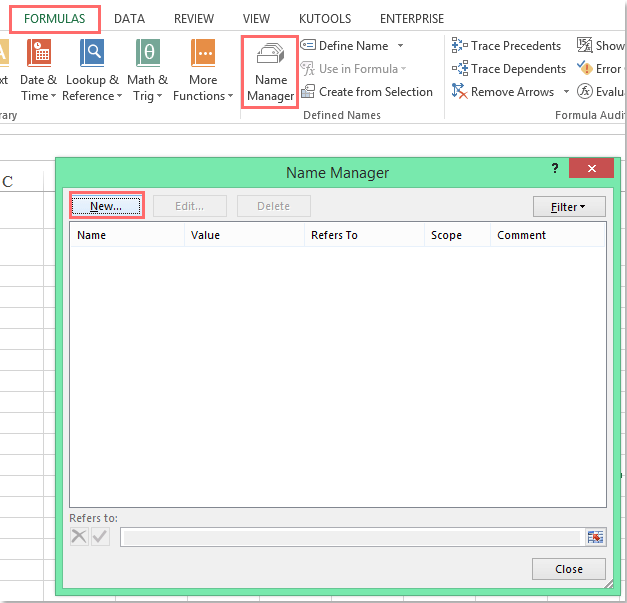2. In the New Name dialog box, in the Name text box, please enter rng as the range name, and then enter this formula: =ROW(INDIRECT(Sheet1!\$B\$1&":"&Sheet1!\$B\$2)) (Sheet1 is your current worksheet you used, B1 and B2 are the start and end numbers you specified )into the Refers to text box, then click OK button to return to the former dialog. See screenshot: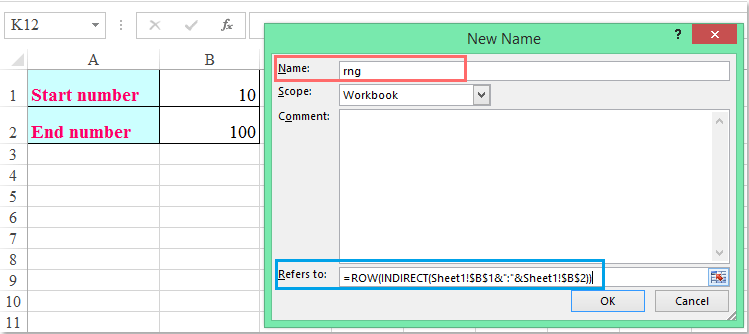3. Go on clicking New button to create another range name, in the New Name dialog box, enter a name prime into the Name text box, and then enter this formula: =SMALL(IF(MMULT(--(IF(rng>TRANSPOSE(rng-Sheet1!\$B\$1+2),MOD(rng,(rng>TRANSPOSE(rng-Sheet1!\$B\$1+2))*TRANSPOSE(rng-Sheet1!\$B\$1+2)))=0),rng-Sheet1!\$B\$1+2)=0,rng),ROW(INDIRECT("1:"&Sheet1!\$B\$2))) (rng is the first range name you have created in step2) into the Refers to text box, see screenshot: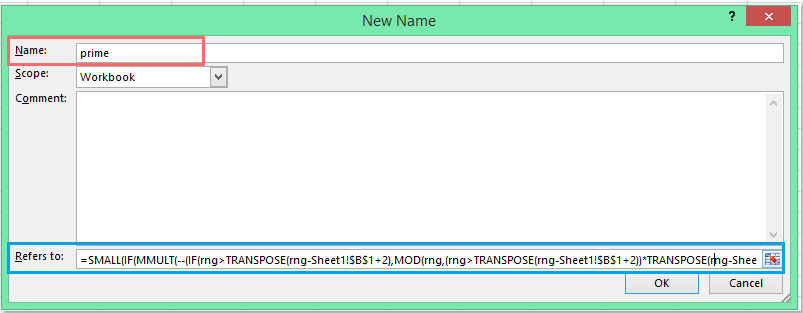4. Then click OK and close the dialogs, and select one column that you want to list all the prime numbers, and enter this formula: =IFERROR(prime,"")(prime is the range name you created in step 3) into the formula bar, and then press CTRL + SHIFT + ENTER keys together, all the prime numbers between the given two numbers are listed as following screenshot shown: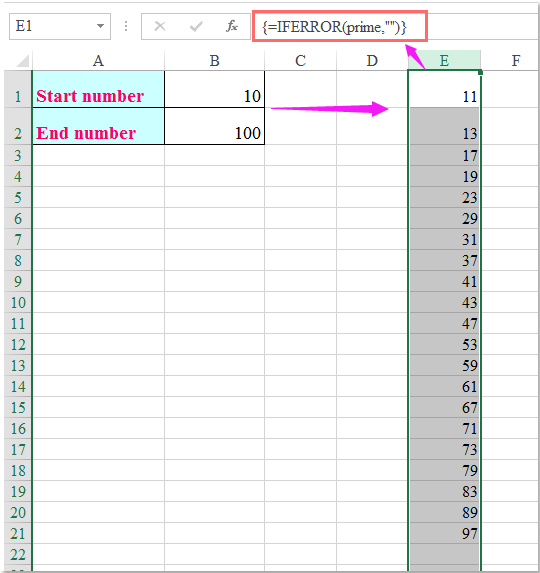####Generate all prime numbers between two given numbers with User Defined Function

If the first method is somewhat difficult, here, you can apply a User Defined Function to solve it.

1. Hold down the ALT + F11 keys to open the Microsoft Visual Basic for Applications window.

2. Click Insert > Module, and paste the following code in the Module Window.

VBA code: Generate all prime numbers between two specific numbers:

```Function PRIME(St, En As Long)
'Updateby Extendoffice 20160613
Dim num As String
For n = St To En
For m = 2 To n - 1
If n Mod m = 0 Then GoTo 20:
Next m
num = num & n & ","
20:
Next n
PRIME = num
End Function
```

3. Then save and close this code, go back to the worksheet, please enter this formula: =prime(10,100) (10 is the start number, and 100 is the end number that you want to get the prime numbers between, you can change them to your need), and then press Enter key, and all the prime numbers are displayed into one cell, see screenshot: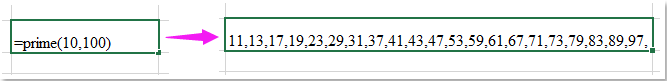### The Best Office Productivity Tools

#### Kutools for Excel Solves Most of Your Problems, and Increases Your Productivity by 80%

• Reuse: Quickly insert complex formulas, charts and anything that you have used before; Encrypt Cells with password; Create Mailing List and send emails...
• Super Formula Bar (easily edit multiple lines of text and formula); Reading Layout (easily read and edit large numbers of cells); Paste to Filtered Range...
• Merge Cells/Rows/Columns without losing Data; Split Cells Content; Combine Duplicate Rows/Columns... Prevent Duplicate Cells; Compare Ranges...
• Select Duplicate or Unique Rows; Select Blank Rows (all cells are empty); Super Find and Fuzzy Find in Many Workbooks; Random Select...
• Exact Copy Multiple Cells without changing formula reference; Auto Create References to Multiple Sheets; Insert Bullets, Check Boxes and more...
• Extract Text, Add Text, Remove by Position, Remove Space; Create and Print Paging Subtotals; Convert Between Cells Content and Comments...
• Super Filter (save and apply filter schemes to other sheets); Advanced Sort by month/week/day, frequency and more; Special Filter by bold, italic...
• Combine Workbooks and WorkSheets; Merge Tables based on key columns; Split Data into Multiple Sheets; Batch Convert xls, xlsx and PDF...
• More than 300 powerful features. Supports Office/Excel 2007-2019 and 365. Supports all languages. Easy deploying in your enterprise or organization. Full features 30-day free trial. 60-day money back guarantee.#### Office Tab Brings Tabbed interface to Office, and Make Your Work Much Easier

• Enable tabbed editing and reading in Word, Excel, PowerPoint, Publisher, Access, Visio and Project.
• Open and create multiple documents in new tabs of the same window, rather than in new windows.
• Increases your productivity by 50%, and reduces hundreds of mouse clicks for you every day!Say something here...
symbols left.
###### or post as a guest, but your post won't be published automatically.
• To post as a guest, your comment is unpublished.
· 2 years ago
starting_number = 1 'input value here
last_number = 10000 'input value here
For a = starting_number To last_number
c = a
For b = 2 To c
If a Mod b = 0 And c b Then
Exit For
Else
If b = c Then
Exit For
Else
If Round(a / (b + 1)) + 1 > b Then
c = Round(a / (b + 1)) + 1
End If
End If
End If
Next b
Next a
End Sub

For a = starting_number To last_number
c = a
For b = 2 To c
If a Mod b = 0 And c b Then
Exit For
Else
If b = c Then
Exit For
Else
If Round(a / (b + 1)) + 1 > b Then
c = Round(a / (b + 1)) + 1
End If
End If
End If
Next b
Next a
End Function

Sub ISPRIME3()
number_to_be_checked = 15485863 'input value here
c = number_to_be_checked
For b = 2 To c
If number_to_be_checked Mod b = 0 And c b Then
MsgBox "Not Prime. Divisible by " & b
Exit Sub
Else
If b = c Then
MsgBox "Prime"
Exit Sub
Else
If Round(number_to_be_checked / (b + 1)) + 1 > b Then
c = Round(number_to_be_checked / (b + 1)) + 1
End If
End If
End If
Next b
End Sub
• To post as a guest, your comment is unpublished.
· 2 years ago
starting_number = 1 'input value here
last_number = 2000 'input value here
For a = starting_number To last_number
For b = 2 To a
If a - b * Int(a / b) = 0 And a b Then
Exit For
Else
If a = b Then
End If
End If
Next b
Next a
End Sub

For a = starting_number To last_number
For b = 2 To a
If a - b * Int(a / b) = 0 And a b Then
Exit For
Else
If a = b Then
End If
End If
Next b
Next a
End Function

'to check if a number is prime
Sub ISPRIME()
number_to_be_checked = 102 'input value here
For b = 2 To number_to_be_checked
If number_to_be_checked - b * Int(number_to_be_checked / b) = 0 And _
number_to_be_checked b Then
MsgBox "Not Prime. Divisible by " & b
Exit Sub
Else
If number_to_be_checked = b Then
MsgBox "Prime"
End If
End If
Next b
End Sub

'to check if a number is prime
Function ISPRIME2(number_to_be_checked)
For b = 2 To number_to_be_checked
If number_to_be_checked - b * Int(number_to_be_checked / b) = 0 And _
number_to_be_checked b Then
ISPRIME2 = "Not Prime. Divisible by " & b
Exit Function
Else
If number_to_be_checked = b Then
ISPRIME2 = "Prime"
End If
End If
Next b
End Function